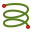# Helix

Create a helix to be used either as a building block for constructing geometry or as a wire.

On the Construct tab, in the Create Arc group, click theHelix icon.
Tip: Press A,4 to use the shortcut key.Origin (C) The centre point of the helix base. Base radius (Rb) The radius of the helix base (parallel to the UV plane). End radius (Rt) The height of the helix, in the N axis direction. Turns (N) The number of turns of the helix (the rotation direction is selected based on the Left handed check box).Origin (C) The centre point of the helix base. Radius (R) The radius of the helix (parallel to the UV plane). Pitch angle (A) The angle formed between the tangent of the curve and the UV plane - constant along the length of the helix. Turns (N) The number of turns of the helix (the rotation direction is selected based on the Left handed check box).Origin (C) The centre point of the helix base. Radius (R) The radius of the helix (parallel to the UV plane). Height (H) The height of the helix, in the N axis direction. Pitch angle (A) The angle formed between the tangent of the curve and the UV plane - constant along the length of the helix.